Function Repository Resource:

# RandomCircleChord

Generate a random chord of a circle

Contributed by: Sander Huisman
 ResourceFunction["RandomCircleChord"][type] returns a random chord of type type. ResourceFunction["RandomCircleChord"][type,n] returns n random chords of type type. ResourceFunction["RandomCircleChord"][type,n,format] returns n random chords of type type with the output format format.

## Details

ResourceFunction["RandomCircleChord"][] returns a single chord of the type: "TwoPointsOnCircle".
ResourceFunction["RandomCircleChord"][All] returns the list of possibles types type.
The type can be any of the following: "TwoPointsOnCircle", "TwoPointsInCircle", "PointInCircleAngle", "RadiusAngleMidpoint", "PointInCircleMidpoint", "CenterAngle":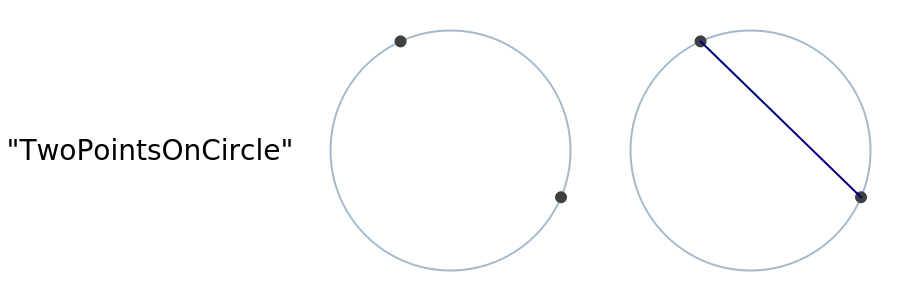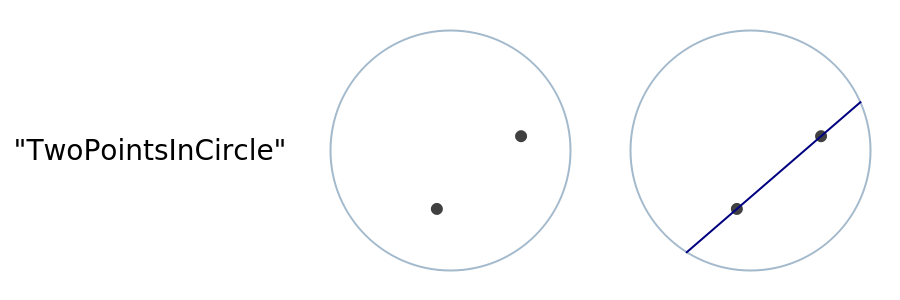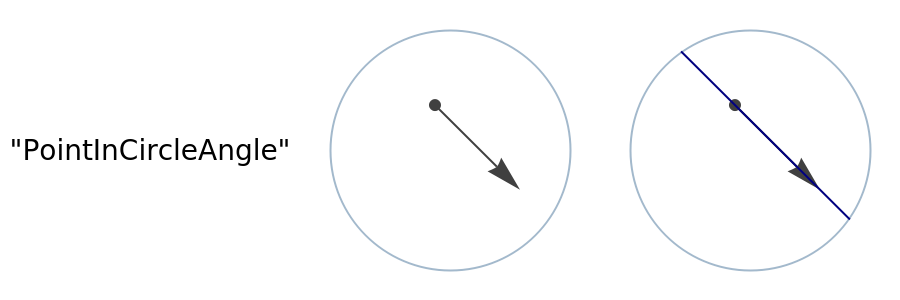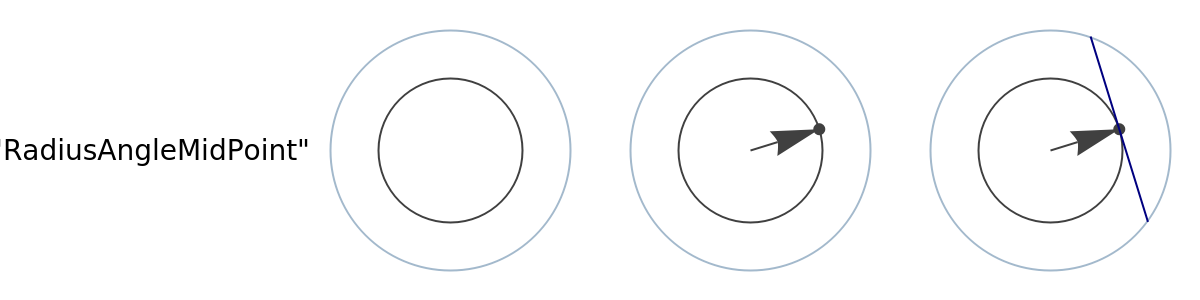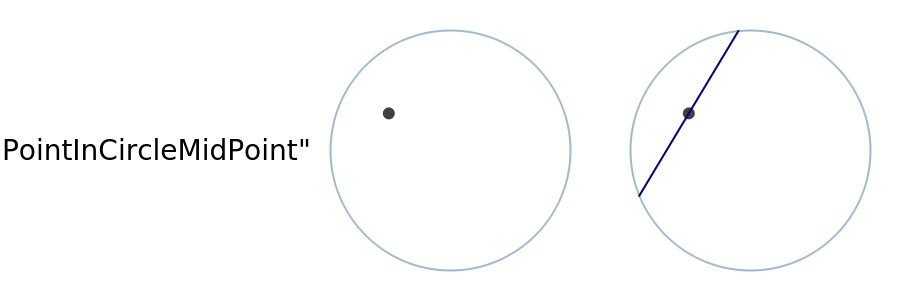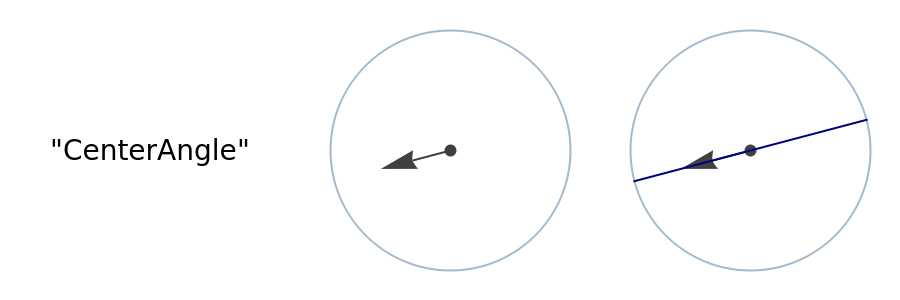The format can be any of the following: "EndPoints", "Line", "Lines", "Midpoints".

## Examples

### Basic Examples (2)

Generate a random circle chord:

 In:=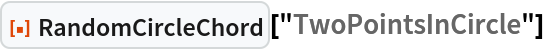Out=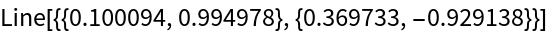Generate 100 random circle chords and visualize:

 In:=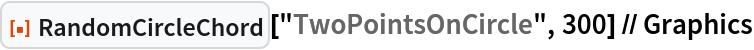Out=### Scope (6)

Retrieve all types:

 In:=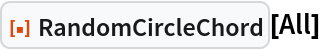Out=By default, the chords are returns as a list of Line objects::

 In:=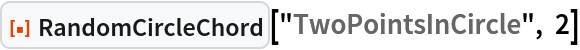Out=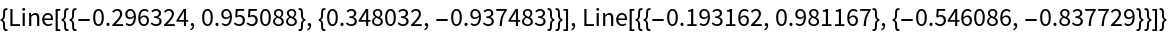Get the endpoints of the chord:

 In:=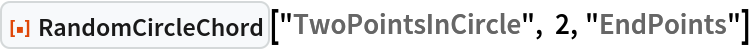Out=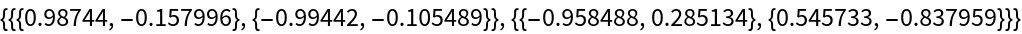Represent the chords as a single Line object:

 In:=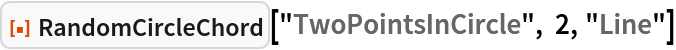Out=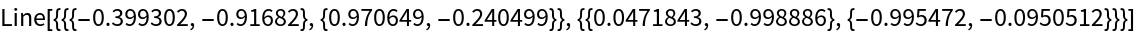Get the midpoints of each chord:

 In:=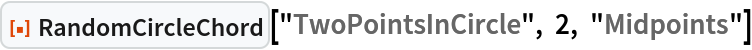Out=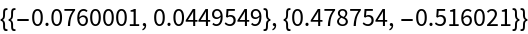Generate 50 chords and return a single Line object:

 In:=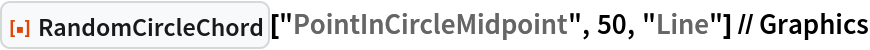Out=### Applications (1)

Find the chance of each slice of a pie getting a candle given that the two candles are randomly placed and the cake randomly cut:

 In:=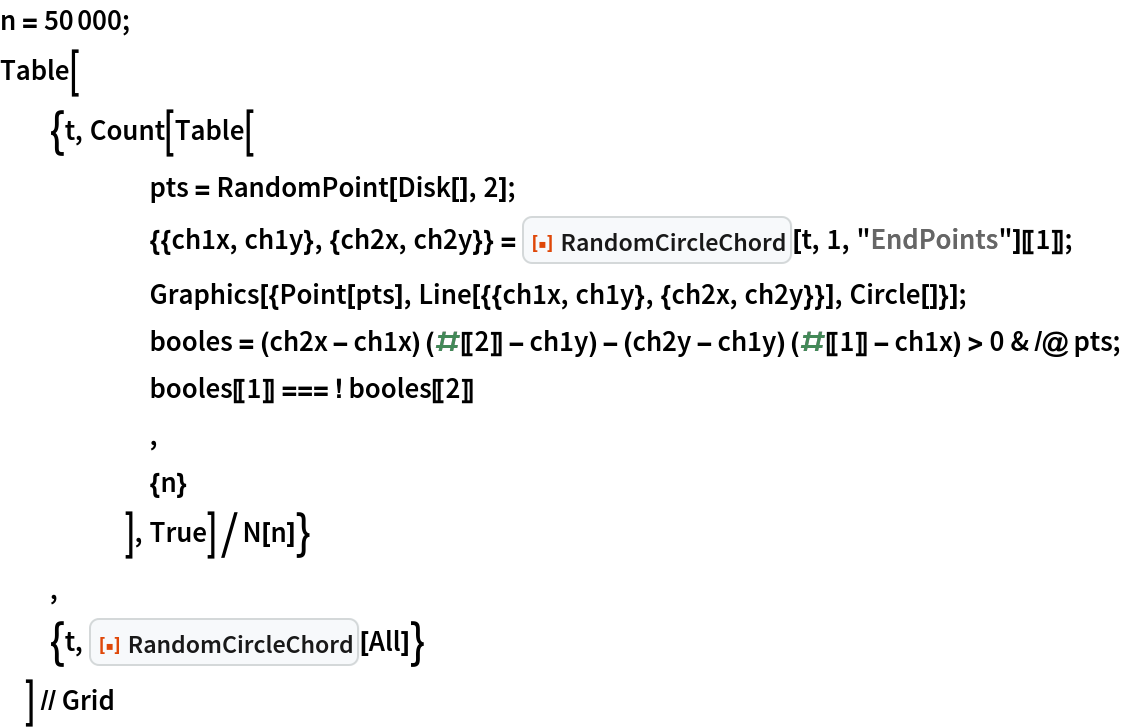Out=### Properties and Relations (1)

Compare the distribution of midpoints visually:

 In:=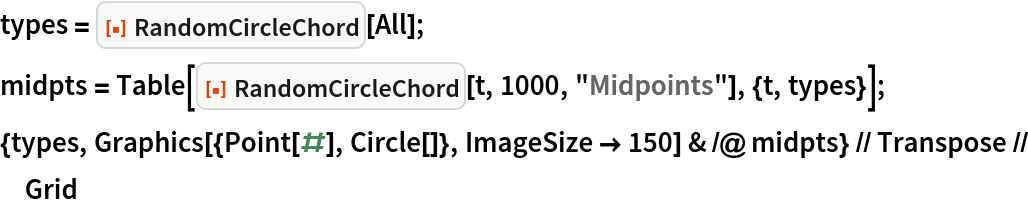Out=### Neat Examples (2)

Find the radial distribution of the midpoints:

 In:=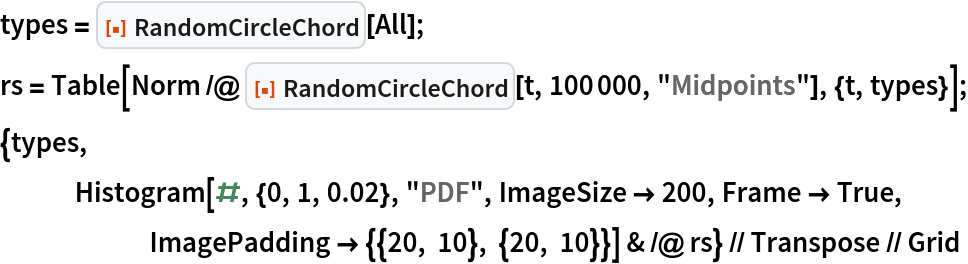Out=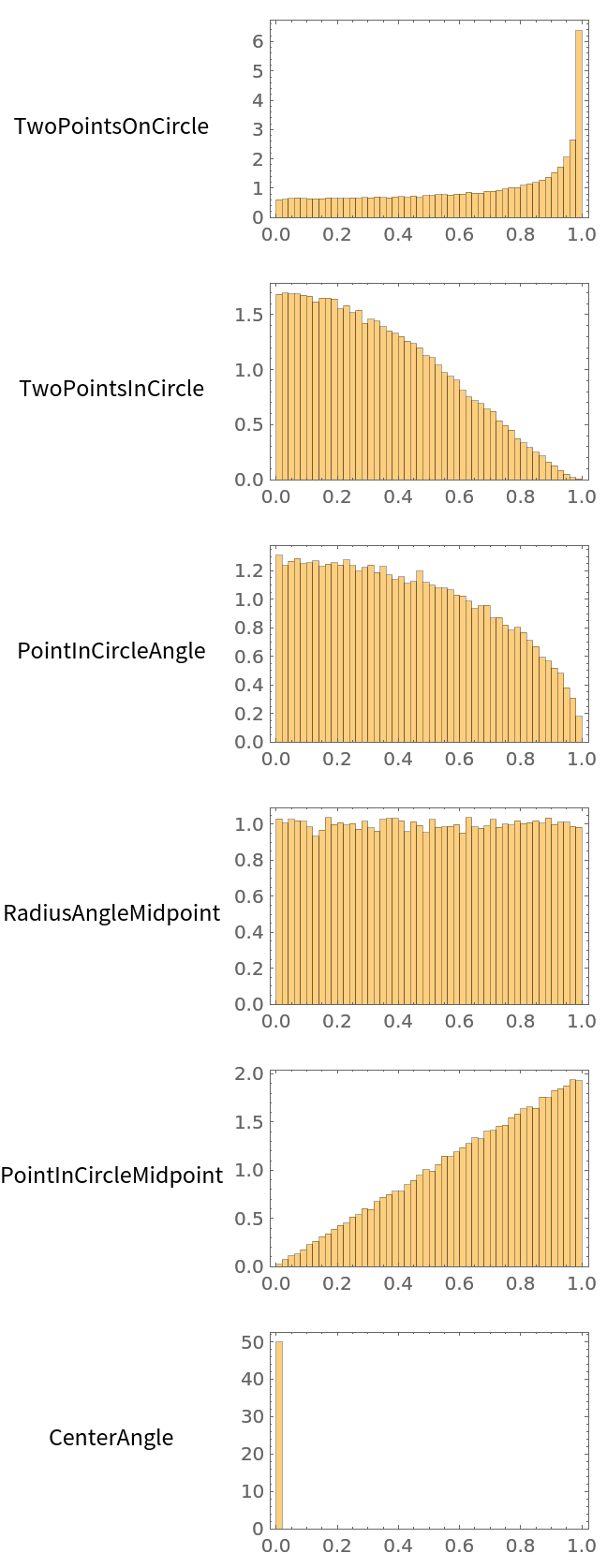Check the Bertrand paradox for a variety of ways of generating random chords:

 In:=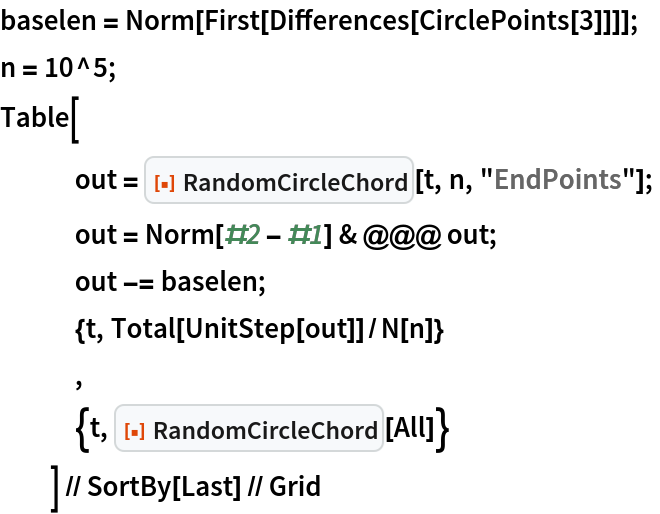Out=SHuisman

## Version History

• 1.0.0 – 17 May 2022